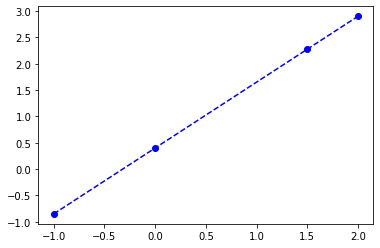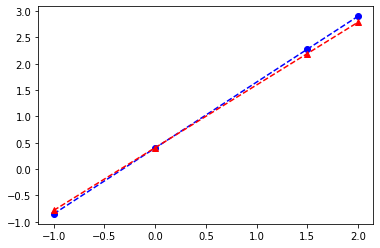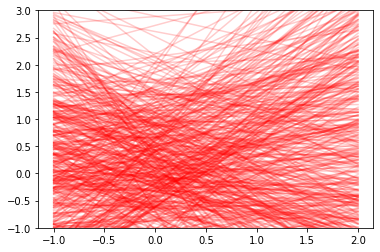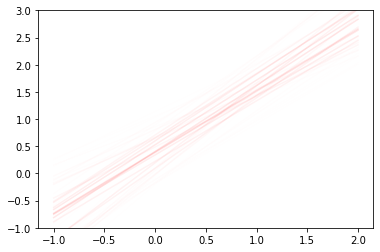### DS4420: Estimation via sampling and LDA¶

Some inspiration here from Iain Murray.

Consider the simple regression example below. We will assume we observe ($x, y$) data from this and want to recover the parameters. Obviously, we could use MLE here, but we will use this to introduce sampling methods.

In :
b0_true = 0.40
b1_true = 1.25

f = lambda x : b0_true + b1_true * x
X = [-1, 0, 1.5, 2.0]
y = [f(x_i) for x_i in X]

In :
from matplotlib.pyplot import plot
import seaborn as sns

plot(X, y, 'bo--')

Out:
[<matplotlib.lines.Line2D at 0x7fb3b83e8080>]Of course, we wouldn't know the true line in practice; the idea is to estimate $b_0$ and $b_1$. Obviously for simple linear regression we can do this via least-squares, but for illustration let's think about using MCMC. Specifically we'll take a Bayesian view and use importance sampling to find parameters.

We might assume the following conditional distribution, given true parameters $\beta$:

$p(y|\beta, x) \sim \mathcal{N}(\beta_0 + \beta_1 \cdot x, \sigma^2)$

And then

$p(\beta) \sim \mathcal{N}([0, 0], I\cdot[\sigma^2_{\text{prior}}, \sigma^2_{\text{prior}}])$

In :
import numpy as np
import scipy

# pick a prior over our coefficients.
# Note: Is this a good choice? Why or why not?
mu_prior, std_prior = *2, *2
prior = scipy.stats.multivariate_normal(mu_prior, std_prior)
prior.pdf([0, 0])

Out:
0.15915494309189535
In :
def candidate_beta():
return np.random.normal(mu_prior, std_prior)


What's the probability of a given $\beta$?

In :
beta = candidate_beta()
y_hat = beta + beta * X
scipy.stats.norm(y, 0.1).pdf(y_hat) * prior.pdf(beta)

Out:
0.0009526407323445032
In :
def data_likelihood(beta, X, y, error_var=.5):
d_prob = 1
# note: am being verbose and inefficient; much better
# to take a matrix vector product
for x_i, y_i in zip(X, y):
y_hat = beta + beta * x_i
d_prob *= scipy.stats.norm(y_i, error_var).pdf(y_hat)
return d_prob


In :
data_likelihood(beta, X, y)

Out:
3.970204507689646e-37
In :
def importance_sample_betas(n_samples):
# sample betas from our prior
samples = [candidate_beta() for _ in range(n_samples)]

# calculate data likelihood -- p(y|X,\beta) and multiply by prior for
# all betas
likelihoods = [data_likelihood(beta, X, y) * prior.pdf(beta) for beta in samples]

# normalize the probabilities
z = sum(likelihoods)
weights = [l/z for l in likelihoods]

# take a weighted average over samples
#import pdb; pdb.set_trace()
return (np.dot(np.array(samples).T, np.array(weights)), samples, weights)

In :
# [0.4, 1.25]
beta_est, betas, weights = importance_sample_betas(500)
print(beta_est)
plot(X, y, 'bo--')
y_hat = [beta_est + x_i * beta_est for x_i in X]
plot(X, y_hat, 'r^--')

[0.40619106 1.19020783]

Out:
[<matplotlib.lines.Line2D at 0x7fb408a9a3c8>]Let's look at the samples we drew.

In :
import matplotlib.pyplot as plt

f, ax = plt.subplots()
for beta_hat, w in (zip(betas, weights)):
y_hat = [beta_hat + x_i * beta_hat for x_i in X]
ax.plot(X, y_hat, 'r-', alpha=0.2)
ax.set_ylim((-1,3))
f.show()

/anaconda3/lib/python3.7/site-packages/ipykernel_launcher.py:8: UserWarning: Matplotlib is currently using module://ipykernel.pylab.backend_inline, which is a non-GUI backend, so cannot show the figure.And now let's factor in their weights. What's happening here is that most of the lines are so unlikely that they don't appear at all!

In :
f, ax = plt.subplots()
for beta_hat, w in (zip(betas, weights)):
y_hat = [beta_hat + x_i * beta_hat for x_i in X]
ax.plot(X, y_hat, 'r-', alpha=w)
ax.set_ylim((-1,3))
f.show()

/anaconda3/lib/python3.7/site-packages/ipykernel_launcher.py:6: UserWarning: Matplotlib is currently using module://ipykernel.pylab.backend_inline, which is a non-GUI backend, so cannot show the figure.## Coming back to LDA¶

OK, so as we said, this is overkill for regression -- there are (much) better ways for estimating these parameters. We started out motivated by estimating parameters in LDA.

$\beta_k \sim {\text{Dirichlet}}(\eta)$

$\theta_d \sim {\text{Dirichlet}}(\alpha)$

$z_{dn} \sim \text{Discrete}(\theta_d)$

$x_{dn}|z_{dn} \sim \text{Discrete}(\beta_k)$

Unfortunately, this is a much more complicated model, and naive importance weighting will not work. However, similar ideas will! In particular, we can use MCMC methods -- namely Gibbs, which we will next review.

### Implementation (credit to Mathieu Blondel)¶

Below is a nice implementation of the collapsed Gibbs model we reviewed from Mathieu Blondel (original at https://gist.github.com/mblondel/542786#file-lda_gibbs-py-L8).

In [ ]:
"""
(C) Mathieu Blondel - 2010

Implementation of the collapsed Gibbs sampler for
Latent Dirichlet Allocation, as described in

Finding scientifc topics (Griffiths and Steyvers)
"""

import numpy as np
import scipy as sp
from scipy.special import gammaln

def sample_index(p):
"""
Sample from the Multinomial distribution and return the sample index.
"""
return np.random.multinomial(1,p).argmax()

def word_indices(vec):
"""
Turn a document vector of size vocab_size to a sequence
of word indices. The word indices are between 0 and
vocab_size-1. The sequence length is equal to the document length.
"""
for idx in vec.nonzero():
for i in xrange(int(vec[idx])):
yield idx

def log_multi_beta(alpha, K=None):
"""
Logarithm of the multinomial beta function.
"""
if K is None:
# alpha is assumed to be a vector
return np.sum(gammaln(alpha)) - gammaln(np.sum(alpha))
else:
# alpha is assumed to be a scalar
return K * gammaln(alpha) - gammaln(K*alpha)

class LdaSampler(object):

def __init__(self, n_topics, alpha=0.1, beta=0.1):
"""
n_topics: desired number of topics
alpha: a scalar (FIXME: accept vector of size n_topics)
beta: a scalar (FIME: accept vector of size vocab_size)
"""
self.n_topics = n_topics
self.alpha = alpha
self.beta = beta

def _initialize(self, matrix):
n_docs, vocab_size = matrix.shape

# number of times document m and topic z co-occur
self.nmz = np.zeros((n_docs, self.n_topics))
# number of times topic z and word w co-occur
self.nzw = np.zeros((self.n_topics, vocab_size))
self.nm = np.zeros(n_docs)
self.nz = np.zeros(self.n_topics)
self.topics = {}

for m in xrange(n_docs):
# i is a number between 0 and doc_length-1
# w is a number between 0 and vocab_size-1
for i, w in enumerate(word_indices(matrix[m, :])):
# choose an arbitrary topic as first topic for word i
z = np.random.randint(self.n_topics)
self.nmz[m,z] += 1
self.nm[m] += 1
self.nzw[z,w] += 1
self.nz[z] += 1
self.topics[(m,i)] = z

def _conditional_distribution(self, m, w):
"""
Conditional distribution (vector of size n_topics).
"""
vocab_size = self.nzw.shape
left = (self.nzw[:,w] + self.beta) / \
(self.nz + self.beta * vocab_size)
right = (self.nmz[m,:] + self.alpha) / \
(self.nm[m] + self.alpha * self.n_topics)
p_z = left * right
# normalize to obtain probabilities
p_z /= np.sum(p_z)
return p_z

def loglikelihood(self):
"""
Compute the likelihood that the model generated the data.
"""
vocab_size = self.nzw.shape
n_docs = self.nmz.shape
lik = 0

for z in xrange(self.n_topics):
lik += log_multi_beta(self.nzw[z,:]+self.beta)
lik -= log_multi_beta(self.beta, vocab_size)

for m in xrange(n_docs):
lik += log_multi_beta(self.nmz[m,:]+self.alpha)
lik -= log_multi_beta(self.alpha, self.n_topics)

return lik

def phi(self):
"""
Compute phi = p(w|z).
"""
V = self.nzw.shape
num = self.nzw + self.beta
num /= np.sum(num, axis=1)[:, np.newaxis]
return num

def run(self, matrix, maxiter=30):
"""
Run the Gibbs sampler.
"""
n_docs, vocab_size = matrix.shape
self._initialize(matrix)

for it in xrange(maxiter):
for m in xrange(n_docs):
for i, w in enumerate(word_indices(matrix[m, :])):
z = self.topics[(m,i)]
self.nmz[m,z] -= 1
self.nm[m] -= 1
self.nzw[z,w] -= 1
self.nz[z] -= 1

p_z = self._conditional_distribution(m, w)
z = sample_index(p_z)

self.nmz[m,z] += 1
self.nm[m] += 1
self.nzw[z,w] += 1
self.nz[z] += 1
self.topics[(m,i)] = z

# FIXME: burn-in and lag!
yield self.phi()

if __name__ == "__main__":
import os
import shutil

N_TOPICS = 10
DOCUMENT_LENGTH = 100
FOLDER = "topicimg"

def vertical_topic(width, topic_index, document_length):
"""
Generate a topic whose words form a vertical bar.
"""
m = np.zeros((width, width))
m[:, topic_index] = int(document_length / width)
return m.flatten()

def horizontal_topic(width, topic_index, document_length):
"""
Generate a topic whose words form a horizontal bar.
"""
m = np.zeros((width, width))
m[topic_index, :] = int(document_length / width)
return m.flatten()

def save_document_image(filename, doc, zoom=2):
"""
Save document as an image.

doc must be a square matrix
"""
height, width = doc.shape
zoom = np.ones((width*zoom, width*zoom))
# imsave scales pixels between 0 and 255 automatically
sp.misc.imsave(filename, np.kron(doc, zoom))

def gen_word_distribution(n_topics, document_length):
"""
Generate a word distribution for each of the n_topics.
"""
width = n_topics / 2
vocab_size = int(width ** 2)

m = np.zeros((n_topics, vocab_size))

for k in range(width):
m[k,:] = vertical_topic(width, k, document_length)

for k in range(width):
m[k+width,:] = horizontal_topic(width, k, document_length)

m /= m.sum(axis=1)[:, np.newaxis] # turn counts into probabilities

return m

def gen_document(word_dist, n_topics, vocab_size, length=DOCUMENT_LENGTH, alpha=0.1):
"""
Generate a document:
1) Sample topic proportions from the Dirichlet distribution.
2) Sample a topic index from the Multinomial with the topic
proportions from 1).
3) Sample a word from the Multinomial corresponding to the topic
index from 2).
4) Go to 2) if need another word.
"""
theta = np.random.mtrand.dirichlet([alpha] * n_topics)
v = np.zeros(vocab_size)
for n in range(length):
z = sample_index(theta)
w = sample_index(word_dist[z,:])
v[w] += 1
return v

def gen_documents(word_dist, n_topics, vocab_size, n=500):
"""
Generate a document-term matrix.
"""
m = np.zeros((n, vocab_size))
for i in xrange(n):
m[i, :] = gen_document(word_dist, n_topics, vocab_size)
return m

if os.path.exists(FOLDER):
shutil.rmtree(FOLDER)
os.mkdir(FOLDER)

width = N_TOPICS / 2
vocab_size = width ** 2
word_dist = gen_word_distribution(N_TOPICS, DOCUMENT_LENGTH)
matrix = gen_documents(word_dist, N_TOPICS, vocab_size)
sampler = LdaSampler(N_TOPICS)

for it, phi in enumerate(sampler.run(matrix)):
print ("Iteration", it)
print ("Likelihood", sampler.loglikelihood())

if it % 5 == 0:
for z in range(N_TOPICS):
save_document_image("topicimg/topic%d-%d.png" % (it,z),
phi[z,:].reshape(width,-1))